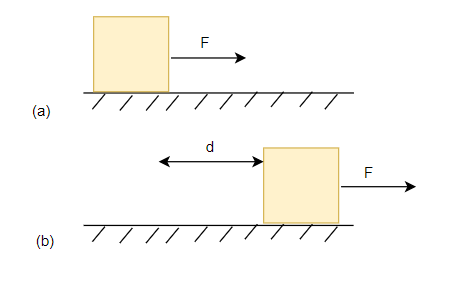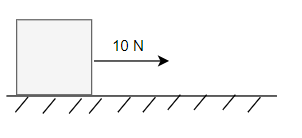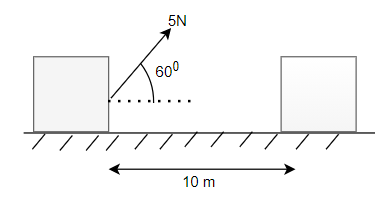# Work Calculator

Note
• Enter the values of the two known variables in the text boxes
• Leave the text box empty for the variable you want to solve for
• Click on the calculate button.

Formula used
$\tau=Fd$

## What is Work

In physics, we define work as the product of the magnitude of applied force and the distance through which the body moves.
.Here we take the applied force which is acting along the line of directionWork is given by
$W=Fd$
where
F-> Force Acting on the Body in the direction of displacement
d ->displacement of the object
W -> Work done by the Force
. So if the force is acting at an angle to the displacement,we take the component of force along the direction of displacement for Work Calculation Work is a Scalar quantity . The SI unit of Work is Joule
It can be expressed in vector form as the scalar product of force and displacement
$W = \mathbf{F} . \mathbf{d}$
Where
$\mathbf{F}$ is the force vector acting on the particle
$\mathbf{d}$ is the displacement vector of the object

Example of Few questions where you can use this Work Calculator
Question 1
A Force of 10N is acting on a body and body moves by 10m in below figure.What is the workdone by the ForceFind the Work acting
Solution
Now we can see from the figure force is acting along the direction of displacement.So, F=10 N
d=10 m
Now as per Work formula
$W=Fd = 10 \times 10 = 100 \ J$

Question 2
A Force of 5N is acting on a body and body moves by 10m in below figure.What is the workdone by the ForceSolution
Now we can see from the figure force is acting at angle 60° the direction of displacement.So, F=5 cos 60= 2.5 N
Now d=10 m
Now as per force formula
$W=Fd = 2.5 \times 10 = 25 \ J$

## How the Work Calculator works

1. If force and distance are given, Work is calculated as
$W= Fd$
2. If force and Work are given, distance is calculated as
$d= \frac {W}{F}$
3. If Work and distance are given, force is calculated as
$F = \frac {W}{d}$